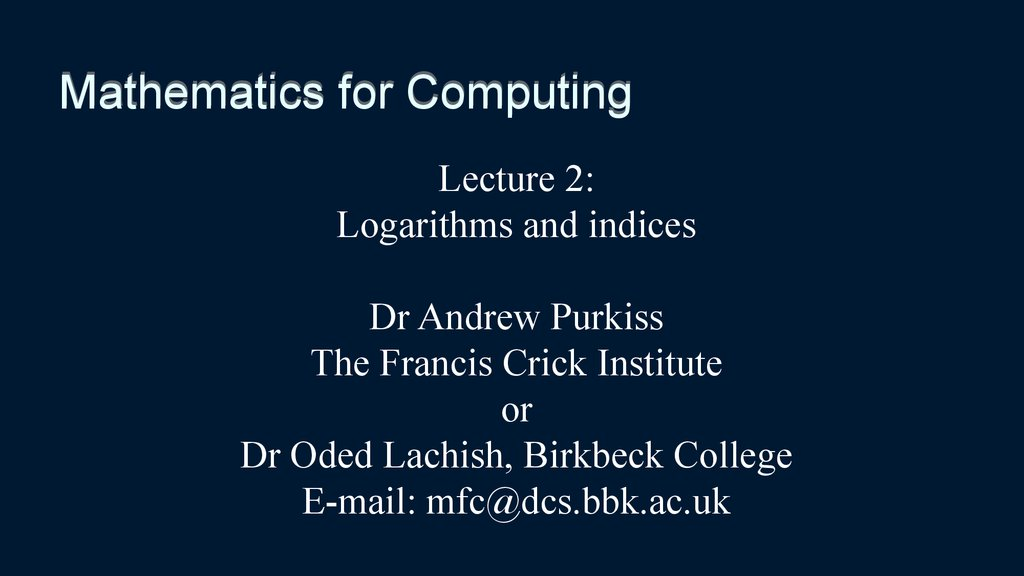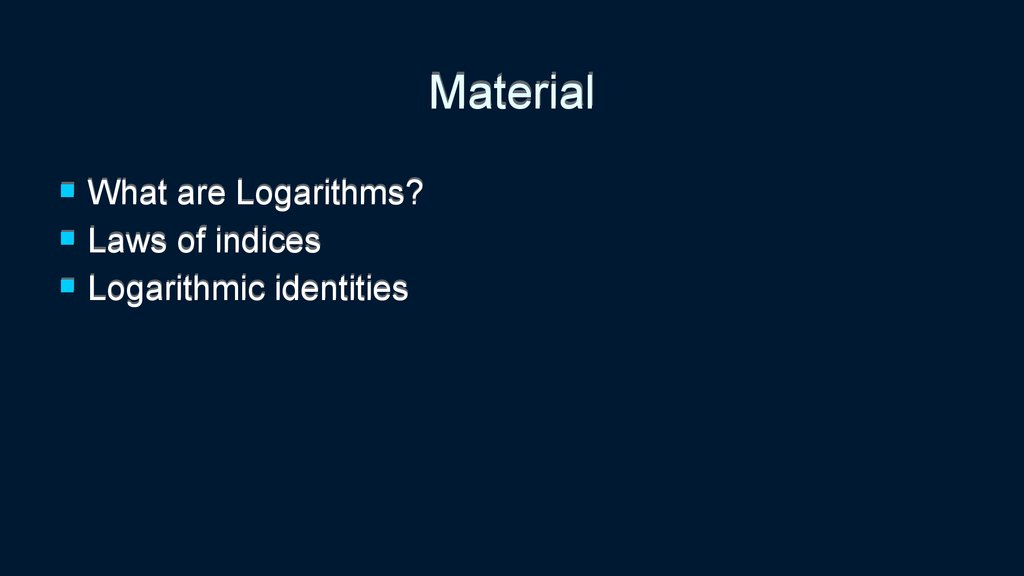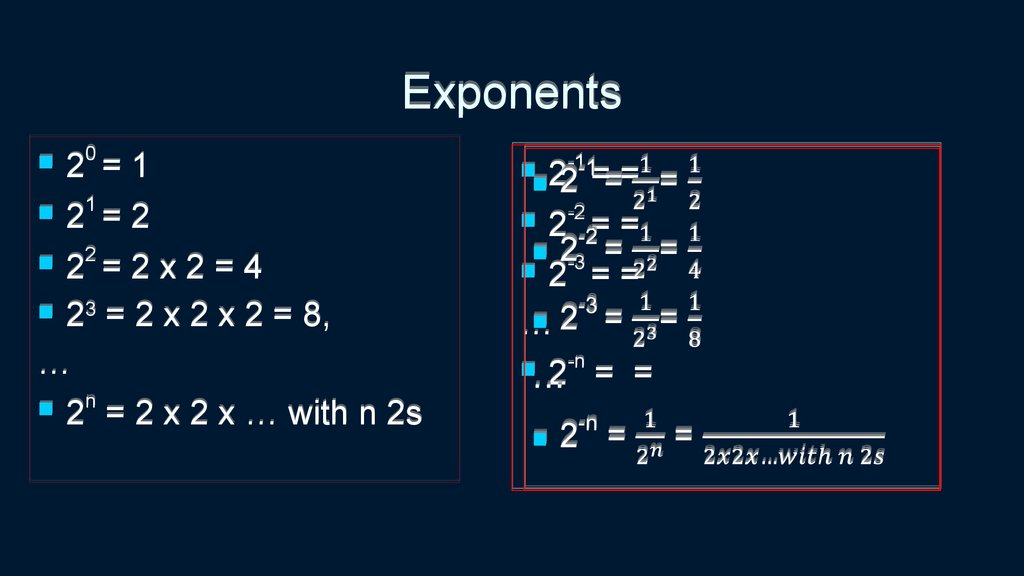# Mathematics for Computing. Lecture 2: Logarithms and indices

## 1. Mathematics for Computing

Lecture 2:
Logarithms and indices
Dr Andrew Purkiss
The Francis Crick Institute
or
Dr Oded Lachish, Birkbeck College
E-mail: [email protected]

## 2. Material

What are Logarithms?
Laws of indices
Logarithmic identities

## 3. Exponents

0
2 =1
1
2 = 2
2
2 = 2 x 2 = 4
23 = 2 x 2 x 2 = 8,

n
2 = 2 x 2 x … with n 2s
-1
2 ==
-2
2 = =
2-3 = =

2-n = =

## 4. Problem

We want to know how many bits the
number 456 will require when stored in
(non signed) binary format.
Solution based on what we learned last
week: Convert the number to Binary and
count the number of bits
After counting we get 9 (check it out)
There is a simpler way
Digit
numb
er
Numb
er
Remainder
when
dividing by 2
1
2
3
4
5
6
7
8
9
456
228
114
57
28
14
7
3
1
0
0
0
1
0
1
1
1
1

## 5. A simpler way

Round 456 up to the smallest power of 2 that is greater than 456.
Specifically, 512.
9
Notice that 512 = 2 .
Why did we round up?
index
9
8
7
6
5
4
3
2
1
0
1
1
0
1
0
1
0
1
0
0
0
1
0
0
0
0
0
0
0
456
512
29
This gives us 2 to the power of the 1 + the index of the MSB of our number,
which is 1 less than its number of bits because the indices start from 0!

## 6. A simpler way

Much better, but we really don’t like the rounding up to the
smallest …
Don’t worry we just did this specific rounding up so that the
We will show a simpler way to do this (although we will start with
512 since it is nicer)

## 7. Logarithms

If we already knew the 512, then we would wonder which number is such
that
2x = 512
In words, how many times do we need to multiply 2 by itself to get 512?
The formal way to write this is x = log2512 , which means how many times do
we need to multiply 2 by itself to get 512?
This is interpreted as follows:

## 8. Logarithms

We only know 456, lets compute log base 2 of 456
log2456 = 8.861…
Rounding this number up gives the answer we wanted, 9!
Why didn’t we get an integer? Because 456 is not a power of 2 so to get 456
we need to multiply 2 by itself 8.861 times, which can be done once we know
what this means.
So, how many bits do need in order to store the number 3452345 in binary
format?

If x = yz
then z = logy x

## 10. Logarithms and Exponents

If x = yz
then z = logy x
e.g. 1000 = 10 3,
then 3 = log10 (1000)
The base

## 11. Logarithms and Exponents: general form

From lecture 1) base index form:
number = baseindex
then index = logbase (number)

x^2
x^3
10
30
9
20
8
7
10
6
5
X^2
X^3
0
-4
4
-3
-2
-1
0
-10
3
2
-20
1
-4
-3
-2
-1
0
0
x
1
2
3
4
-30
x
1
2
3
4

Log10
Log2
4
2
0
Logn(x)
0
2
4
6
-2
-4
-6
-8
x
8
10
12

10^x
10^x
100000
100000
80000
10000
60000
1000
10^x
-5
10^x
-4
-3
-2
-1
40000
100
20000
10
0
0
x
1
2
3
4
5
-5
-4
-3
-2
-1
1
0
x
1
2
3
4
5

## 15. Three ‘special’ types of logarithms

Common Logarithm: base 10
Common in science and engineering
Natural Logarithm: base e (≈2.718).
Common in mathematics and physics
Binary Logarithm: base 2
Common in computer science

1) a0 = 1
2) a1 = a

1) a0 = 1
2) a1 = a
Examples:
20 = 1
100 = 1

1) a0 = 1
2) a1 = a
Examples:
21 = 2
101 = 10

3) a-x = 1/ax

## 20. Laws of indices

3) a-x = 1/ax
Example:
3-2 = 1/32 = 1/27

## 21. Laws of indices

4) ax · ay = a(x + y)
(a multiplied by itself x times) · (a multiplied by itself y times) = a multiplied by itself x+y times
5) ax / ay = a(x - y)
(a multiplied by itself x times) divided by (a multiplied by itself y times) = a multiplied by itself x-y times

## 22. Laws of indices

4) ax · ay = a(x + y)
42 · 43 = 4(2+3) = 45
16x64 = 1024
9 · 27 = 32 · 33 = 3(3 + 2) = 35= 243
25 · (1/5) = 52 · 5-1 = 5(2-1) = 51= 5

## 23. Laws of indices

5) ax / ay = a(x - y)
105 / 103 = 10(5-3) = 102
100,000 / 1,000 = 100
23 / 27 = 2(3-7) = 2-4
8 / 128 = 1/16, [24 = 16, 2-4 = 1/16, see law 3)]
64 / 4 = 26 / 22 = 2(6- 2) = 24 = 16
27 / 243 = 33 / 35 = 3(3 - 5) = 3-2= 1/9
25 / (1/5) = 52 / 5-1 = 5(2+1) = 53= 125

## 24. Laws of indices

6)
(ax)y = axy
(a multiplied by itself x times) multiplied by itself y times) = a multiplied by itself x ·y times
X times
X times
X times
(a ·a ·…) ·(a ·a ·…) ·…(a ·a ·…)
y times
7)
ax/y =
y
a1/y is the number you need to multiply by itself y times to get a. (a1/y)y = ay/y = a1 =a
1/2
1/3
So , 2 is square root of 2, which is, and 3 is square root of 3, which is,

## 25. Laws of indices

6) (ax)y = axy
(103)2 = 10(3x2) = 106
1,0002 = 1,000,000
(24)2 = 2(2x4) = 28
162 = 28 = 256
81 = (9) 2 = (32)2 = 34 = 81
1/16 = (1/4) 2 = (2-2)2 = 2-4 = 1/16

## 26. Laws of indices

7) ax/y = y√ax
10(4/2) = 2√104
102 = 2√10,000 = 100
2(9/3) = 3√29
23 = 3√512 = 8
8 = 23 = 26/2 = 2√64 = 8
1/7 = (7) -1 = (7) -2/2 = 2√(1/49) = 7

‘Trivial’
Log form
logb 1 = 0
logb b = 1
Index form
b0 = 1
b1 = b

## 28. Logarithmic identities 2

y · logb x = logb xy
Definition of log
(bx)y = bxy
Definition of log

## 29. Logarithmic identities 2 examples

y · logb x = logb xy
(bx)y = bxy
Examples:
9 = 3 · log 8 = log 83 = log 512 = 9
2
2
2
512= (8)3 = (23)3 = 23·3= 29 = 512

## 30. Logarithmic identities 3

Negative Identity
-log x = log (1/x)
b
b
b-x = 1/bx
log x + log y = log xy
b
b
b
bx · by = b(x + y)
Subtraction
log x - log y = log x/y
b
b
b
bx / by = b(x - y)

## 31. Negative Identity

(3rd law of indices)
(definition of log)
Taking log from both
sides of the equation
(definition of log)

## 32. Negative identity

Negative Identity
-log x = log (1/x)
b
b
b-x = 1/bx
Examples:
-3 = -log 8 = log (1/8) = -3
2
2
1/8 = 2-3 = 1/23 =1/8

bx · by = b(x + y) (4th law of indices)
Taking log from
both sides of the
equation
Definition of log
(definition of log)

log x + log y = log xy
b
b
b
bx · by = b(x + y)
Examples:
5= 2+3 = log 4 + log 8 = log 4·8 = log 32 = 5
2
2
2
2
32= 4 · 8 = 22 · 23 = 2(2 + 3) = 25 = 32

## 35. Subtraction Identity

b ·b =b
x
y
(x + y)
(4 law of indices)
th
Taking log from
both sides of the
equation
Definition of log
(definition of log)
(definition of log + 3rd law of
indices )

## 36. Subtraction identity examples

Subtraction
log x - log y = log x/y
b
b
b
bx / by = b(x - y)
Examples:
-1 = 2-3 = log 4 - log 8 = log 4/8 = log 1/2 = -1
2
2
2
2
1/2= 4 / 8 = 22 / 23 = 2(2 - 3) = 2-1 = 1/2
3 = 5-2 = log2 32 - log2 4 = log2 32/4 = log2 8 = 3
8= 32 / 4 = 25 / 22 = 2(5 - 2) = 23 = 8

## 37. Changing the base

logb x = logy x / logy b
logb x = 1/(logx b)

## 38. Changing the base, examples 1

logb x = logy x / logy b
Examples:
2 = log4 16 = log2 16 / log2 4 = 4/2= 2
4 = log3 81 = log5 81 / log5 3

## 39. Changing the base, examples 2

logb x = 1/(logx b)
Examples:
2 = log 16 = 1/log 4 = 1/(1/2)= 2
4
16
4 = log3 81 = 1/ log81 3
= 1/(1/4)= 4